# Voltage Divider: What is it? (Circuit And Applications)

Contents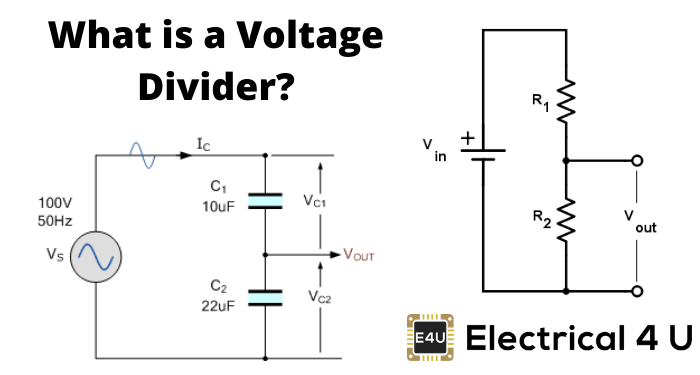## What is a Voltage Divider?

A voltage divider is a fundamental circuit in the field of electronics which can produce a portion of its input voltage as an output. It is formed using two resistors (or any passive components) and a voltage source. The resistors are connected in series here and the voltage is given across these two resistors.

This circuit is also termed as a potential divider. The input voltage is distributed among the resistors (components) of the voltage divider circuit. As a result, the voltage division takes place. If you’re looking for help on the calculation for voltage division, you can use our voltage divider calculator.

## Circuit of Voltage Divider

As we mentioned above, two series resistors and voltage source constitute a simple voltage divider. This circuit can be formed in several ways as shown below.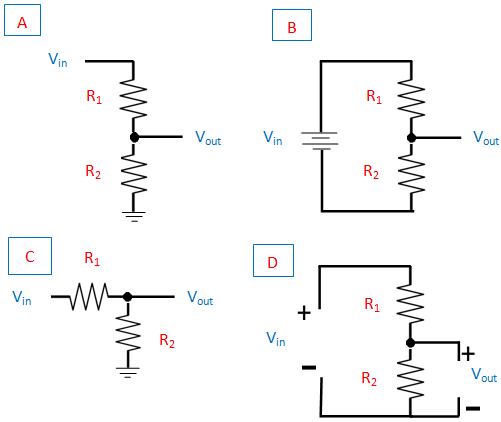In the above figure, (A) represents shorthand, (B) represents longhand and (C) and (D) shows the resistors in different and same angle respectively.

But all the four circuits are in effect the same. R1 is the resistor that is always close to the input voltage source and R2 is the resistor that is near to the ground. Vout is the voltage drop across the resistor, R2.

It is actually the divider voltage that we get from this circuit as the output.

### Equation of Voltage Divider in Unloaded Condition

The simple voltage divider circuit with reference to the ground is shown in the figure below. Here, two electrical impedances (Z1 and Z2) or any passive components are connected in series. The impedances may be resistors or inductors or capacitors.

The output of the circuit is taken across the impedance, Z2.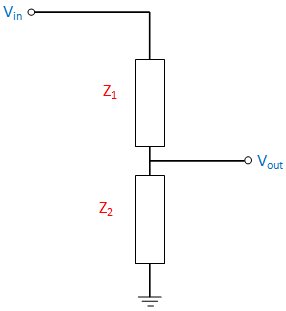Under open-circuit output condition; that is there will be no current flow in the output side, then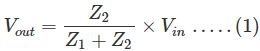Now we can prove the output voltage equation (1) using the basic law, Ohm’s Law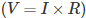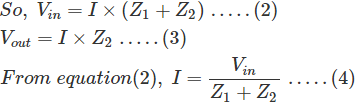Substitute equation (4) in (3), we get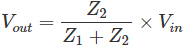So, the equation is proved.
The transfer function of the above equation is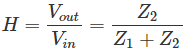This equation is also called as Divider’s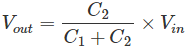The capacitive divider circuits never allow DC input to pass. They work on AC input.

For an Inductive divider with non-interacting inductors, the equation becomes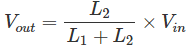The inductive divider divides the DC input analogous to resistor divider circuit depending on resistance and it divides AC input with regard to the inductance.

A basic low-pass RC filter circuit is shown below which comprises of a resistor and capacitor.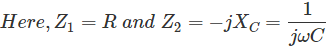C → Capacitance
R → Resistance
XC → Reactance of the capacitor
ω → Radiant frequency
j → Imaginary unit
Here, the divider’s voltage ratio is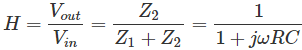RC → Time constant of the circuit represented as τ.

## Voltage Divider Under Loaded Condition

Now, we can see the voltage divider circuit in a loaded condition. Here, the resistors (R1 and R2) are taken for simplicity. A resistor (RL) is connected across the output. Then the equation becomes,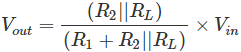R2 and RL are parallel to each other.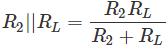The circuit with the loaded condition is shown below.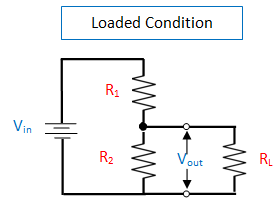## Applications of Voltage Divider

Applications include Logic level shifting, Sensor measurement, High voltage measurement, Signal Level Adjustment.

The measuring instruments such as Multimeter and Wheatstone bridge consist of the voltage divider.

The resistor voltage divider is usually used to generate reference voltages or for decreasing the magnitude of the voltage for ease of measurement.

In addition to this; at low frequency, it can function as signal attenuators.

In the case of DC and very low frequencies, the resistor voltage divider is suitable. The capacitive voltage divider is implemented in power transmission for high voltage measurement and to compensate load capacitance.

Want To Learn Faster? 🎓
Get electrical articles delivered to your inbox every week.
No credit card required—it’s 100% free.